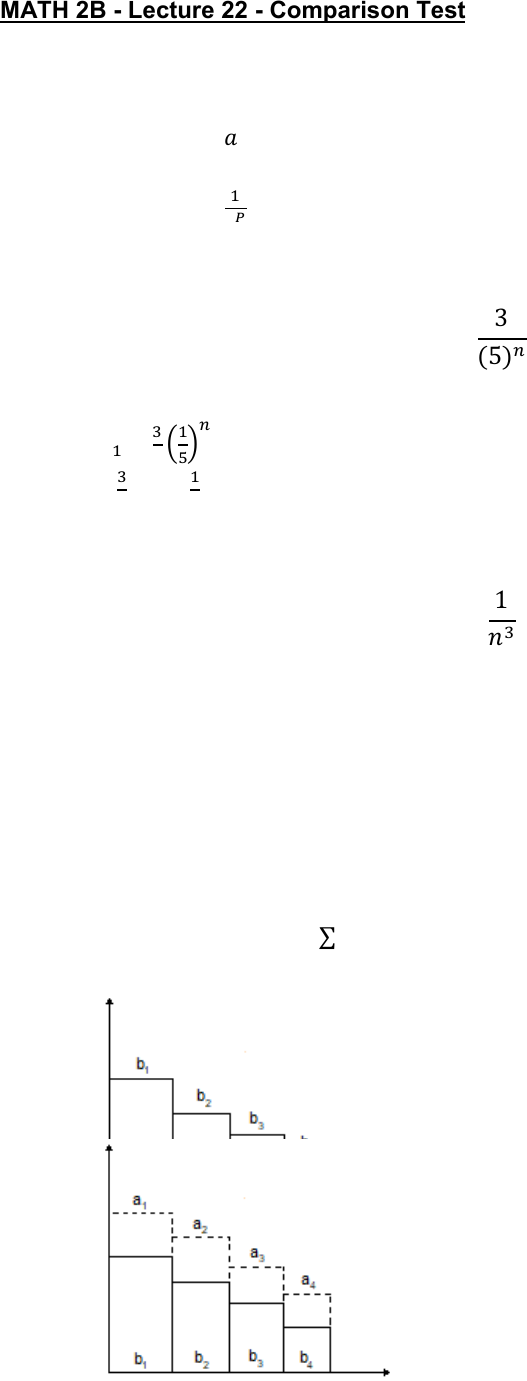Class Notes (1,100,000)
US (460,000)
UC-Irvine (10,000)
MATH (1,000)
MATH 2B (600)
Lecture 22

# MATH 2B Lecture 22: Comparison TestPremium

Department
Mathematics
Course Code
MATH 2B
Professor
ERJAEE, G.
Lecture
22

This preview shows half of the first page. to view the full 3 pages of the document.02/25/2019
MATH 2B - Lecture 22 - Comparison Test
We start from two important series
1. Geometric series
      
2. P-series

    
Example:

Solution:



converges
Example:

Solution:
   converges
The Comparison Test
Suppose that
 and
 have positive terms
We can consider
 and
 as sum of rectangles, with positive
height
1. If
 converges, and  
 also converges
• If each term of
 is smaller than that of
 then

  
• Converges if
 converges

also
 diverges and  
 2. If
also diverges
• If  


 diverges →
